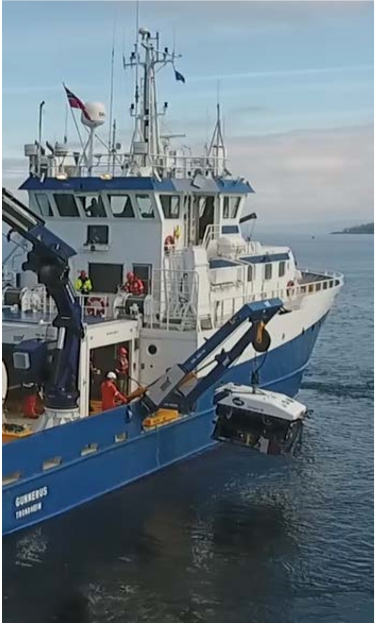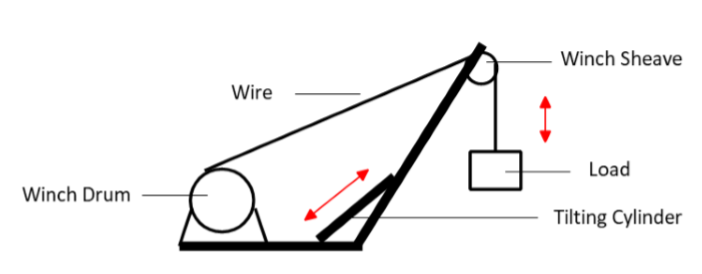# Launch and Recovery System

## System Description

The launch and recovery system (LARS) consist of two main subsystems, i.e., the A-Frame and the winch, as shown in Figure 1. Figure 2 depicts the modeling sketch of the LARS. The LARS model of interest has two degrees of freedom (DoF) enabled by the tilting cylinder and the winch drum through the sheave connected to the A-Frame beam.Figure 1: The LARS on Gunnerus vessel in operation launching the ROV (Photo credit. NTNU)Figure 2: *Modelling sketch of the LARS

## Subsystems, Submodels and FMUS

The reference system model is divided into eight sub-models and FMUs as shown in Figure 3

Table 1: List of FMUs in the LARS system.

FMU name Input Output Default Parameters
WinchActuatorSetpoint   winchSetpoint
WinchController `winchSetpoint`, `loadSpeed` `motorGain` Proportional gain K = 10.
Derivative time constant Td = 0.1.
Derivative gain limitation N = 10.
Integral time constant Ti = 0.1.
WinchActuator `motorGain`, `motorSpeed` `motorTorque` Max motor output torque T = 10kNm
Winch `motorTorque1`, `motorTorque2`, `motorTorque3` `motorSpeed`, `loadSpeed`, `winchForce` Gear ratio r = 141
Gear efficiency e = 0.925
Drum inertia i = 1600kgm^2
Drum diameter d = 1.36m
Drum width B = 1.1m
Max wire length wireLmax = 1000m
Wire diameter D= 0.02m
Wire Young’s Modulus E = 85e9
Wire damping critical coefficient c= 0.5
Total friction coefficient r = 1
AFrameActuatorSetpoint   `aFrameSetpoint`
AFrameController `aFrameSetpoint`, `cylinderSpeed` `cylinderGain` Proportional gain K = 2
Derivative time constant Td = 0.01
Derivative gain limitation N = 10
Integral time constant Ti = 0.01
Cylinder min/max/init length 1.2/1.7/1.7m
AFrameActuator `cylinderGain`, `cylinderSpeed` `cylinderForce` Max cylinder output force F = 5000kN
AFrame `cylinderForce`, `winchForce` `cylinderSpeed` tilting leg length l=2m
cylinder positions a = 0.46m, b = 1.3572m

## Default Scenario Setup

The simulated scenario presents the retrieving of the ROV, as shown in Figure 4. Specifically, From 5s to 95s, retrieving the ROV by spooling in the winch drum. From 95s to 100s, by retracing the tilting cylinder the A-Frame operates from overboard position to the onboard position.

Given the default setpoint values in `WinchActuatorSetpoint` and `AFrameActuatorSetpoint` , the tilting angle of the A-Frame and the position of the load are shown in Figure 5. Time step 0.001s.